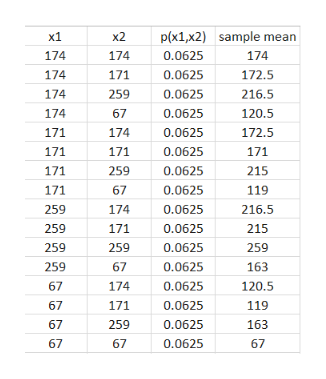# The number of books sold over the course of the four-day book fair were 174, 171, 259, and 67. Assume that samples of size 2 are randomly selected with replacement from this population of four values. Identify the probability of each sample, and describe the sampling distribution of the sample means.

Question
18 views

The number of books sold over the course of the four-day book fair were 174, 171, 259, and 67. Assume that samples of size 2 are randomly selected with replacement from this population of four values. Identify the probability of each sample, and describe the sampling distribution of the sample means.

check_circle

Step 1

Total number of possible samples =4*4 =16 (As 4 choices for each value).

As all sample occur with equal probability, probability of each sample =1/16 (0.0625).

Below is sampling distribution of mean.

...help_outlineImage Transcriptioncloseх1 p(x1,x2) sample mean х2 174 174 0.0625 174 174 171 0.0625 172.5 0.0625 174 259 216.5 174 67 0.0625 120.5 171 174 0.0625 172.5 171 171 0.0625 171 171 259 0.0625 215 171 67 119 0.0625 259 174 0.0625 216.5 171 259 0.0625 215 259 259 259 0.0625 259 67 0.0625 163 67 174 0.0625 120.5 171 67 0.0625 119 67 259 0.0625 163 67 67 0.0625 67 fullscreen

### Want to see the full answer?

See Solution

#### Want to see this answer and more?

Solutions are written by subject experts who are available 24/7. Questions are typically answered within 1 hour.*

See Solution
*Response times may vary by subject and question.
Tagged in

### Other